### Brewer’s PPS Method

Brewer’s method (METHOD=PPS_BREWER) selects two units from each stratum, with probability proportional to size and without replacement. The selection probability for unitin stratumequals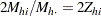. (Because selection probabilities cannot exceed 1, the relative size for each unit,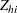, must not exceed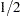.)

Brewer’s algorithm first selects a unit with probability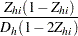where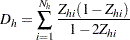Then a second unit is selected from the remaining units with probability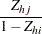where unitis the first unit selected. The joint selection probability for unitsandin stratumequals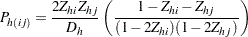See Cochran (1977, pp. 261–263) and Brewer (1963) for details. Brewer’s method yields the same selection probabilities and joint selection probabilities as Durbin’s method. See Cochran (1977) and Durbin (1967) for details.